# Strand7 linear and nonlinear transient dynamic solver

The transient dynamic solver is used to calculate the time history of the dynamic response of a structure subjected to any arbitrary forcing function and initial conditions. Linear dynamic equilibrium equations are in the following form: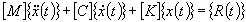where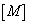Mass matrix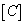Damping matrix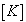Stiffness matrixNodal displacement vector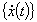Nodal velocity vector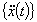Nodal acceleration vector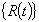Load vector

For the nonlinear transient dynamic solver, the above expression is modified to include the effects of nonlinearities on the equilibrium. As in the nonlinear transient dynamic solver the nonlinear equilibrium equations are always linearised in each time step, the following discussion, based on the above expression is still valid.

Forcing Function

Dynamic loads applied to the model are factored through the use of factor vs time tables, which can be any time history. Loads can be point forces and moments, element loads, body loads or thermal loads. Multiple load cases may be combined to form a single loading condition. Each load case may included, excluded or factored according to a factor vs time table.

Multiple freedom cases may also be included if the full system option is used. The constant terms in the freedom cases may be factored in the same way as the loads in the load cases. This provides support for time dependent displacements.

Acceleration of the base of the model (i.e. the nodes with fixed freedoms) may be specified to define the excitation. The time history can be arbitrary and is specified in the factor vs time table. The excitation may act in any general direction defined through the direction vector.

Initial Conditions

Three types of initial conditions can be specified:
1. A linear static solution which specifies the displacement of a structure initially under certain static loads.

2. A transient solution, which specifies the dynamic response of the structure at a time instance. The solution will start from any selected time step.

3. Initial velocity and acceleration of all free nodes in the structure.

Solution Techniques

Two approaches to transient dynamic solutions are available: mode superposition and full system.
Mode Superposition
Using the mode superposition method, responses of the individual modes of the structure are calculated separately and then combined to produce the total response of the structure. This is only applicable to linear transient dynamic analysis.

In addition to the other advantages of the mode superposition technique, this method offers more in a transient dynamic analysis. One of the advantages is that modal damping can be used in addition to (or instead of) Rayleigh damping. When experimental data are available, modal damping gives a more accurate representation of the damping in the system.

The mode superposition method is best suited to structures where the lower frequencies dominate the response (i.e. earthquakes). Typically, 10 modes will provide good accuracy for these problems. Modal superposition is not suitable for problems such as shock loads or impacts where the higher frequency modes are excited. In these cases, 50 or more modes may be required and the cost to calculate this many modes can significantly offset the saving in the transient solution. The most serious disadvantage of this approach is that it is not capable of handling any nonlinearity in the solution.
Full System
This approach does not have the limitations of mode superposition, but can be computationally very expensive, as all nodal displacements are numerically integrated at the specified time steps. This method is also referred to as direct integration.

The linear transient dynamic solver performs the following steps:
• Initialises the nodal displacement, velocity and acceleration vectors according to the specified initial conditions.

• For mode superposition calculates and assembles equivalent element force vectors and external nodal force vectors. If base acceleration is included, the global mass matrix is also formed for the calculation of the pseudo load vector.

• For direct integration, calculates and assembles element stiffness, mass and damping matrices, equivalent element force vectors and external nodal force vectors. In the stiffness calculation, material temperature dependency is considered. The element geometric stiffness matrix is also included if initial conditions are used. Rayleigh damping and element material damping can be included. Either consistent or lumped element equivalent load vectors can be calculated according to the option setting. Constraints are also assembled in this process, and the constant terms in enforced displacement and shrink links are combined and applied. At the end of this assembly procedure, the three global matrices in the equation of dynamic equilibrium are formed: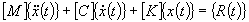• If the full system approach is used, decomposes the global stiffness matrix such that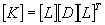• Loops through the specified time steps and calculates displacement, velocity and acceleration using either the Wilson theta or Newmark beta method. When base acceleration is applied, either relative or absolute values of displacement, velocity and acceleration may be calculated.

• Calculates element results such as stress and strain.

The nonlinear transient dynamic solver performs the following steps:
• Initialises the nodal displacement, velocity and acceleration vectors according to the specified initial conditions. For a restart run, all quantities required for describing the current element deformation and/or stress status are recovered from both the initial and the temporary solution file.

• Starts a new time step and calculates the constants required for the time integration if the time stepping is different from the previous one.

• Calculates and assembles the element stiffness matrices, equivalent element force vectors and external nodal force vectors. In the stiffness calculation, material temperature dependency is considered. Depending on whether material and/or geometric nonlinearity is considered, the current material modulus and geometry will be used. The element geometric stiffness matrix is also included if the corresponding option is set. Either consistent or lumped element equivalent load vectors can be calculated according to the option setting. Constraints are also assembled in this process and the constant terms in enforced displacement and shrink links are combined and applied. At the end of this assembly procedure, the global stiffness, mass and damping matrices and nodal force vector are formed.

• Uses the Newmark beta method to calculate the displacement, velocity and acceleration vectors and then updates the current displacement vector.

• Checks for convergence

Displacement norm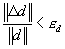Residual force norm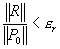where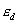and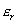are convergence tolerance on displacement and residual force,and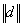are norms of incremental and total displacement vectors,is the norm of the residual force vector at the first iteration of each time step and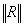is the norm of the residual force vector in the current iteration.

If both of the convergence criteria are satisfied and there are more time steps, the solution continues with the next step otherwise it stops. If either of the criteria is not satisfied, the solution continues the integration and iterates on the current step.

### Notes

1. The results of a transient analysis are provided as series of solutions for discrete points in time throughout the time period of interest. The number and spacing of the result sets can be controlled using the time steps in the solver parameters. The results include the nodal displacements, velocity and acceleration, as well as element stresses, strains and other quantities at that particular time instance.

2. The choice of the correct time step size is important to ensure that the complete response of the structure is captured by the solution and that the solution is stable and free from divergence. This is particularly important for nonlinear solutions.
In general the smaller the time step the more accurate the solution. However, there are practical limits on how small the step can be, as more solution steps will be required for a smaller time step and the running time will normally increase accordingly. Therefore, a limit may be set by the time required for the solution. The size of the time step is also limited by the number of sets of results that can be physically stored. However, the latter limit can be removed in Strand7 by using a periodical saving option.

If the time step is too large then much of the higher frequency response of the structure will be missed and the solution may not adequately represent the real behaviour of the structure as shown below.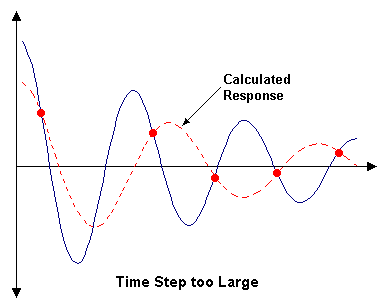For more information on dynamic analysis, see Strand7 Webnotes - Linear / Dynamics and Strand7 Webnotes - Nonlinear / Dynamics.